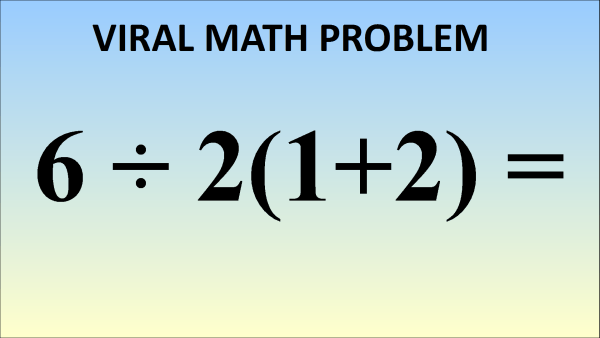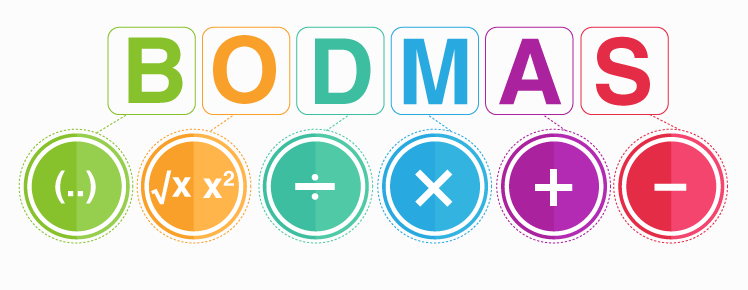# Viral Mathematical Equation Cracking The Heads

1748

Almost everyone hated mathematics for some reasons in their childhood. Today in this tech-savvy world, people are enclosed by the world of social media and even a small viral thing gets escalated quickly. Similarly, a small viral mathematical equation is cracking the head of professional mathematicians and making them bewildered. This equation is –

What is 6÷2(1+2) =?

This mathematical problem is the reason for hot debate and has millions of comments on Facebook, Instagram, Twitter, YouTube, and other platforms of social media sites. People are confused and getting two answers that are 9 and 1. But which one according to you is correct? If you are still confused, then here is the correct solution for this mathematical equation given by experts.• One needs to follow the order of operations. The mathematical equation can be simplified easily by the order of operations, often identified by the acronyms PEMDAS/BODMAS.• First, evaluate Parentheses/Brackets, then evaluate Exponents/Orders, then evaluate Multiplication-Division, and finally evaluate Addition-Subtraction.
• Everyone is in agreement about the first step: simplify the addition inside of the brackets.

6÷2(1+2)
= 6÷2(3)

This is where the debate starts.

The answer is 9

If you type 6÷2(3) into a calculator, Google or sites like WolframAlpha, the input value has to be parsed and then computed. All of these will first change the brackets into an implied multiplication. The equation becomes the following.

6÷2(3)
= 6÷2×3

According to the order of operations, division and multiplication have the same precedence, so the correct order is to evaluate from left to right. First, take 6 and divide it by 2, and then multiply by 3.

6÷2×3
= 3×3
= 9

This gets to the correct answer of 9.This is without argument the correct answer of how to evaluate this expression according to current usage. Some people have a different interpretation. And while it’s not the correct answer today, it would have been regarded as the correct answer 100 years ago.